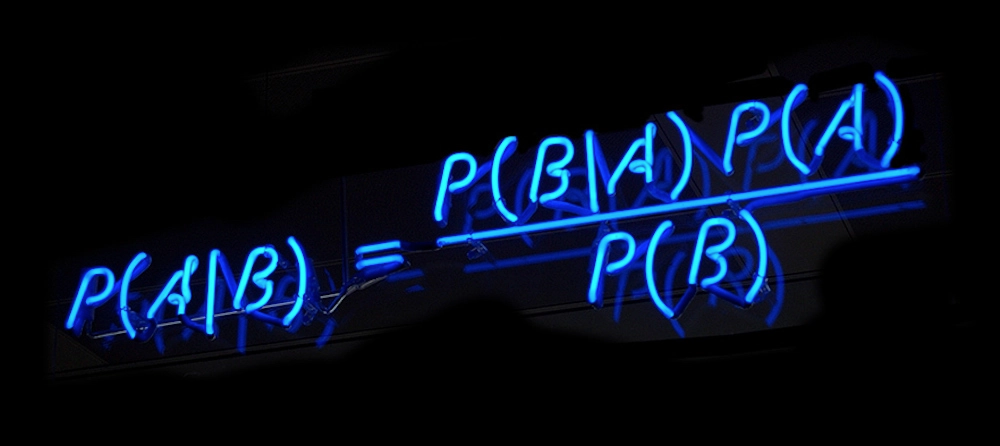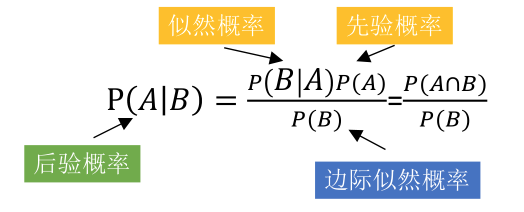﻿# 一、贝叶斯## 什么是先验概率、似然概率、后验概率• 先验概率：男人的老婆没有任何情况，出轨的概率
• 似然概率：男人的老婆出轨了，手机里有暧昧短信的概率
• 后验概率：男人发现老婆手机有暧昧短信，计算他老婆出轨概率

• 先验概率：A厂的产品占产品总数的比例
• 似然概率：A厂的次品率
• 后验概率：已知一件产品是次品，推断这件产品来自在A厂的概率。（次品可能来自ABC中任意一个厂）

## 公式推导• $$P(A|B)$$：表示事件B已经发生的前提下，事件A发生的概率，叫做事件B发生下事件A的条件概率。

• $$P(AB)$$：表示事件A和事件B同时发生的概率

• $$P(AB)=P(BA)$$，可得$$P(AB)=P(BA)=P(B|A)P(A)=P(A|B)P(B)$$

• 通过$$P(A|B)$$来求$$P(B|A)$$，可得$$P(B|A)=\frac {P(A|B)P(B)} {P(A)}$$

• 分母$$P(A)$$可以根据全概率公式分解为：$$P(A)=\sum_{i=1}^n P(B_i)P(A|B_i)$$

• 最终公式变为：$$P(B|A)=\frac {P(A|B)P(B)} {\sum_{i=1}^n P(B_i)P(A|B_i)}$$

# 二、为什么需要朴素贝叶斯$$P(X,Y)=P(Y)⋅P(X∣Y)=P(X)⋅P(Y∣X)$$

• $$P(Y)$$：每个类别出现的概率，先验概率
• $$P(X|Y)$$：给定的类别下不同属性出现的概率，似然概率

# 三、朴素贝叶斯是什么## 条件独立$$P(X=x∣Y=c)=$$

$$P(X(1)=x(1),X(2)=x(2),⋯,X(n)=x(n)∣Y=c)$$

$$\prod_{j=1}^nP(X^j=x^j|Y=c)$$

## 举例：长肌肉## 拉普拉斯平滑## 半朴素贝叶斯半朴素贝叶斯分类器考虑了部分属性之间的依赖关系，既保留了属性之间较强的相关性，又不需要完全计算复杂的联合概率分布。

posted @ 2020-03-30 15:21  雪山飞猪  阅读(...)  评论(...编辑  收藏